# 10. Nonlinear systems

## Introduction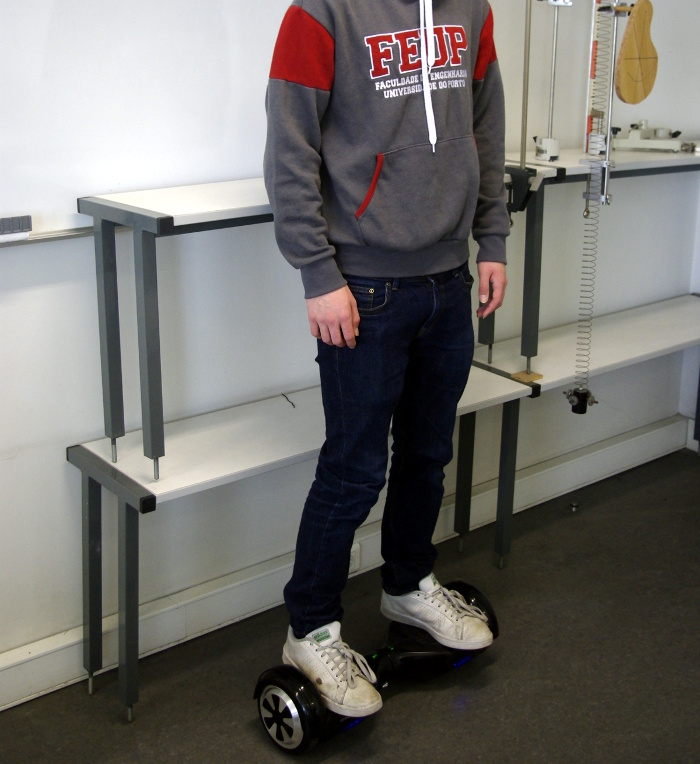A hoverboard has just one axle with two wheels. The person riding it can rotate around that axle as in a pendulum. A pendulum has two equilibrium positions, one where its center of gravity is directly below its axis, and in the other its center of gravity is directly above the axis. The person riding the hoverboard is in that second equilibrium position, which is unstable; if the center of gravity moves slightly out of the vertical that passes through the axle, the weight will make the rider move even further away from that vertical, falling frontwards or backwards. The hoveboard has an automatic control system that makes it move forward or backward, thus bringing the center of gravity back to the equilibrium position. A pendulum like that, oscillating around the stable equilibrium position by means of an external force, is called an inverted pendulum. Other examples of inverted pendulums are a Segway and a mono-cycle.

## 10.1. Linear approximation

Let us consider a continuous dynamical systems with two state variables:

(10.1)

each of the functions and can be expanded as a Taylor series, in the neighborhood of a point ( , ) in phase space:

(10.2)

where the index can be 1 or 2. If the point ( , ) is an equilibrium point, then and, therefore, the first term of the two series is null. Changing the origin of coordinates to the equilibrium point ( , ), that is, in a new coordinate system: , , the first two terms in the series expansion of the functions are,

(10.3)

Which is a linear combination of the new variables and , where the constants multiplying the two variables are the values ​​of the partial derivatives at the equilibrium point ( , ). Substituting these approximations into the system 10.1, we obtain a linear system ( e , because and are constants).

(10.4)

this linear approximation is valid only in a neighborhood of the origin ( , ), namely, for and near the equilibrium point ( , ).

The square matrix in equation 10.4 is called Jacobian matrix, represented here by . Substituting the coordinates ( , ) of the equilibrium point in the Jacobian matrix gives a constant matrix. At each equilibrium point, a different matrix is obtained which defines a linear system that approximates the nonlinear system in a neighborhood of the equilibrium point. The eigenvalues ​​and eigenvectors of each of these matrices can be used to analyze the stability of the system near each equilibrium point, in the same way that it is done for linear systems.

### Example 10.1

Classify the equilibrium points and plot the phase portrait of the system:

Resolution. It has already been shown in the example 7.2 of Chapter 7 that this system has four equilibrium points. Let us save the functions and and the equilibrium points in two Maxima lists:

(%i1) f: [4-x1^2-4*x2^2, x2^2-x1^2+1]\$
(%i2) equilibrio: solve(f)\$

It is also convenient to define another list with the names of the state variables:

(%i3) v: [x1, x2]\$

The jacobian matrix, with two rows and two columns, is obtained using Maxima's command jacobian, which requires two lists, with the functions and the variables names.

(%i4) J: jacobian (f,v);
(%o4)

Substituting the coordinates of each equilibrium point, we obtain the matrices of the linear systems that approximate the nonlinear system in the vicinity of its equilibrium points. For example, at the first equilibrium point,

(%i5) subst (equilibrio, J);
(%o5)

In order to study the stability of the system in the vicinity of this equilibrium point, let us compute the eigenvalues ​​of this matrix.

(%i6) eigenvectors (%)\$
(%i7) float (%);
(%o7)

The result shows 4 lists; the first list are the eigenvalues, the second list are their multiplicities and the last two lists are the eigenvectors corresponding to the two eigenvectors.

At this equilibrium point, the eigenvalues ​​are real, with opposite signs; we then conclude that it is a saddle point. The fourth equilibrium point is also saddle point:

(%i8) subst (equilibrio, J);
(%o8)
(%i9) eigenvectors (%)\$
(%i10) float (%);
(%o10)

At the second equilibrium point we have:

(%i11) subst (equilibrio, J);
(%o11)
(%i12) eigenvectors (%)\$
(%i13) float (map (rectform, %));
(%o13)

Since the eigenvalues ​​are complex, with the negative real part, the equilibrium point is an attractive (stable) focus. Similar calculations for the third equilibrium point show that it is also a focus, but repulsive (unstable), because the real part of the eigenvalues ​​is positive. The phase portrait is created using the command:

(%i14) plotdf (f, v, [x1,-3,3], [x2,-3,3])\$

Figure 10.1 shows the result. There is a single stable equilibrium point, an attractive focus, at ( , ) = (1,265, −0.7746). The other 3 equilibrium points are unstable: two saddle points and a repulsive focus. The two evolution curves shown near the repulsive focus at ( , ) = (−1.265, 0.7746) and their continuation until the saddle points delimit the stability region. When the initial state of the system is in that region, the final state will approach the stable equilibrium point.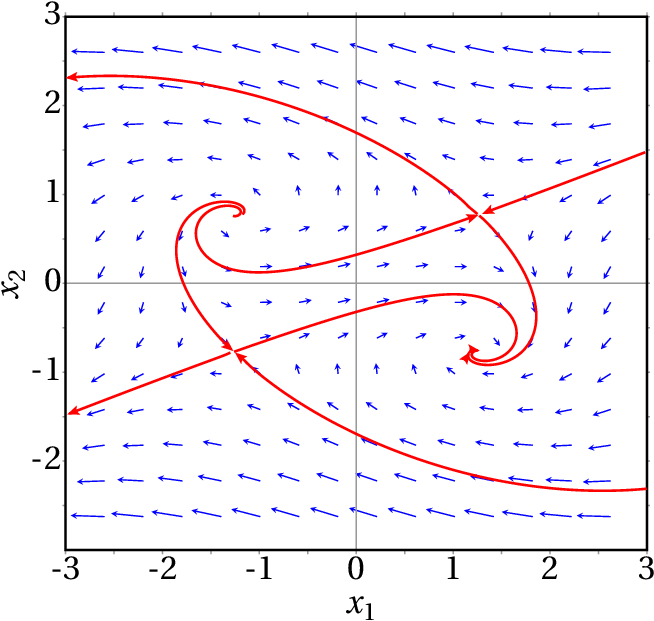Figure 10.1: Phase portrait of the system ˙ x 1 = 4 − x 21 − 4 x 22 , ˙ x 2 = x 22 − x 21 + 1 .

## 10.2. The pendulum

The kind of pendulum that we will study in this section consists of an object connected to a rigid bar crossed by a fixed horizontal axis (Figure 10.2). This type of pendulum can rotate in a vertical plane making complete turns. The system has a single degree of freedom, , which is the angle that the bar makes with the vertical. Let us set when the pendulum is in the lowest position and in its highest position. The angular velocity is and the speed of the center of mass is where is the distance from the center of mass to the axis.

The kinetic energy of the pendulum is:

(10.5)

Where is the total mass and is the moment of inertia with respect to the center of mass. According to the parallel axes theorem 5.23, is the moment of inertia with respect to the axis of the pendulum, which can be written as , where is the radius of gyration relative to the axis. Therefore, the kinetic energy can be written as

(10.6)

The gravitational potential energy is (setting zero energy at )

(10.7)

Ignoring the air resistance, the Lagrange equation leads to the equation of motion:

(10.8)

where defines the effective length of the pendulum. In the particular case of a simple pendulum , in which the mass of the bar is negligible and the disk is very small, equals the distance from the center of the dist to the axis (see example 8.5 in Chapter 8 ).

The evolution equations are obtained by writing the derivative of as the angular velocity and the angular acceleration as the derivative of the angular velocity:

(10.9)

These nonlinear equations can only be solved approximately using some numerical approximation. The Maxima program rk can be used to obtain the numerical solution by the fourth-order Runge-Kutta method. That program requires 4 input parameters: a list with the expressions for the components of the phase velocity, a list with the names of the state variables, a list with initial values ​​for those variables and a range of values ​​for the independent variable, including the name of the variable, its initial value, its final value and the length of the steps to be taken from the initial to the final value. The output of rk is a list of points that approximate the solution; each point will have the coordinates of the independent variable, followed by the values of the state variables at that point.

For example, for a pendulum with effective length of 50 cm, released from rest at an initial angle of 30°, the approximate solution is obtained with the following command ( and stand for and ):

(%i15) s: rk([w,-(9.8/0.5)*sin(q)],[q,w], [%pi/6,0], [t,0,5,0.01])\$

The plots of and vs time and the evolution curve in phase space are obtained with the following commands:

(%i16) plot2d ([[discrete,makelist([p,p],p,s)], [discrete,makelist([p,p],p,s)]], [legend, "angle","angular vel."], [xlabel,"t"]);
(%i17) plot2d ([discrete,makelist([p,p],p,s)], [xlabel,"angle"],[ylabel,"angular vel."]);

The two plots are shown in Figure 10.3.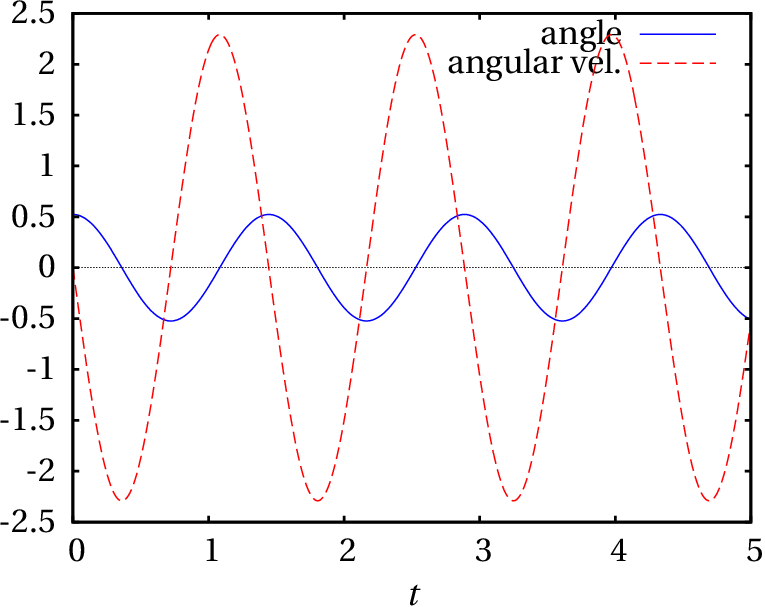Figure 10.3: Oscillations of a 50 cm pendulum with amplitude of 30°.

Looking at the list of values obtained for , one can conclude that the period of oscillation is between 1.44 and 1.45 s. The plots in Figure 10.3 are very similar to the plots for a simple harmonic oscillator. If the initial angle is bigger, this similarity begins to disappear. For example, Figure 10.4 shows the results obtained with an initial angle of 120°.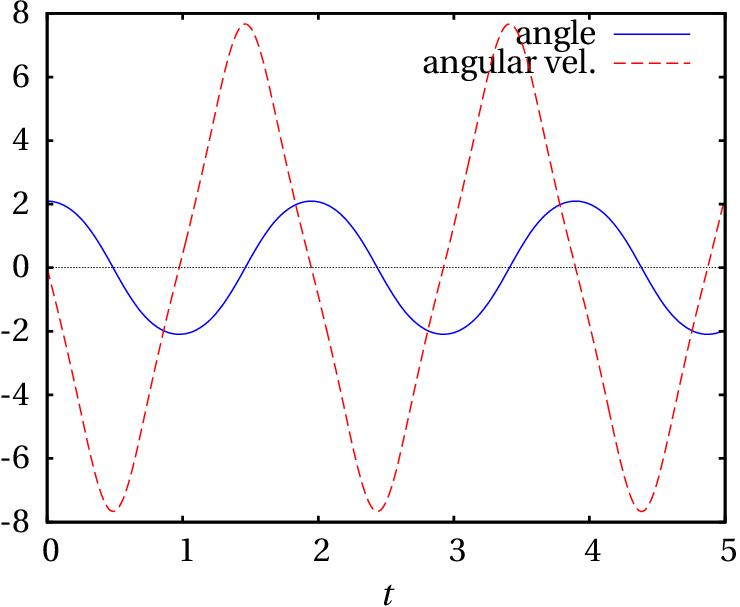Figure 10.4: Oscillations of a 50 cm pendulum with amplitude of 120°.

In this case the period of oscillation is between 1.94 and 1.95 s, which is bigger than in the case of 30° amplitude.

In the two cases shown in Figures 10.3 and 10.4, the evolution curve is a cycle, which implies the existence of a stable equilibrium point inside the cycle.

The equilibrium points of the pendulum, where the right sides of equations 10.9 are zero, occur at , , … and .

The points at , , … are actually the same physical point, corresponding to the pendulum passing through its lowest position after making some complete turns. The points at , … are also the same physical point, at the highest position of the pendulum.

## 10.3. Linear approximation of the pendulum

The Jacobian matrix obtained from the equations 10.9 of the pendulum is

(10.10)

At the equilibrium point in (in general, 0, , ,…), the matrix is:

(10.11)

which is the matrix of a simple harmonic oscillator, discussed in Example 9.4 of Chapter 9. Its two eigenvalues ​​are , so the equilibrium point at is a center and if the initial state of the system is close to that point, the pendulum oscillates with angular frequency . In the case of the 50 cm pendulum considered in the previous section, this expression leads to a period of 1.42 s. Remember that this value is only an approximation, which becomes better the smaller the amplitude; the period values ​​obtained in the previous from a numerical solution of the equations section are more accurate.

In the neighborhood of the equilibrium point at (in general, , ,…), the Jacobian becomes

(10.12)

which is the matrix of an inverted oscillator, discussed in Example 9.3 of Chapter 9. The two eigenvalues ​​are and the equilibrium point is a saddle point (unstable equilibrium).

The phase portrait in the domain will show 3 centers ( , 0 and ) and 4 saddle points ( , , and ). For the cm pendulum we can plot the phase portrait with the command:

(%i18) plotdf([w,-(9.8/0.5)*sin(q)],[q,w],[q,-10,10], [w,-20,20]);

Figure :10.5 shows the result. The angle is in the horizontal axis and the angular velocity is in the vertical axis. The two curves identified with the letters A and B form part of a heteroclinic orbit.

The heteroclinic orbits of the pendulum correspond to the case when the mechanical energy of the pendulum is exactly equal to the gravitational potential energy at the point of maximum height. Since we are using as reference , at the position where the pendulum bar is horizontal ( ), the potential energy at the highest point is . Each of the curves A and B represent the motion starting with the pendulum nearly at rest and very near its highest position, descending and completing a full turn back to the highest position, without further oscillation. The difference between the heteroclinic orbits and cycles is that in cycles the oscillations repeat indefinitely, whereas in a heteroclinic orbit there is a single oscillation.

All the evolution curves in the region of phase space inside the heteroclinic orbit are cycles. Those which are closer to the heteroclinic orbit correspond to oscillations in which the pendulum almost reaches its highest point, it seems to stop there for a few moments and then moves down again to the lowest point, repeating the same motion on the other side of the vertical.

The heteroclinic orbits are also separatrices, because they separate the phase space into a region where the pendulum oscillates —the region shaded in figure 10.6— and a region where the pendulum keeps rotating in the same direction.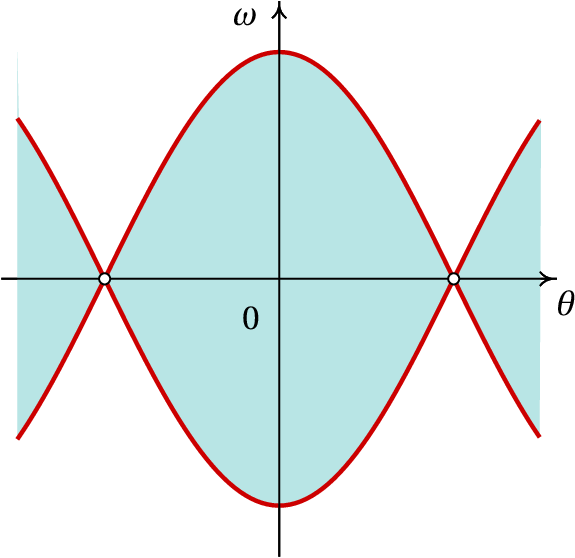Figure 10.6: The heteroclinic orbits delimit the regions where the pendulum oscillates.

Figures 10.3 and 10.4 show that with a 30° amplitude the linear approximation is quite good, since the evolution curve is very similar to that of the simple harmonic oscillator and the period is close to the period obtained with the linear approximation, but with amplitude of 120°, the linear approximation is no longer accurate.

## 10.4. Multi-dimensional phase spaces

In autonomous mechanical systems, for each degree of freedom there is an equation of motion, which implies two state variables. Thus, the dimension of the phase space is twice the number of degrees of freedom. If a system is not autonomous the phase space has one more dimension, as shown in the following section. Thus, the number of dimensions of the phase space for a mechanical systems can be 2, 3, 4, 5, …

In cases when the phase space has more than two dimensions the plotdf program can not be used to show the phase portrait. It is necessary to solve the evolution equations for some specific initial values ​​which can then be used to plot some of the state variables.

### 10.4.1. Systems of non-autonomous equations

The general form of a system with non-autonomous differential equations is:

Each possible state of the system is characterized by the values ​​of the variables and time ; therefore, each state is a point with coordinates ( , ,…, , ) and the phase space has dimensions. The components of the phase velocity are the derivatives of the coordinates of the state: ( , ,…, , ). The expressions for the first components are given by the system of differential equations and the last component is always equal to 1 (derivative of with respect to ). Hence, a non-autonomous system with equations is a dynamical system with state variables.

Such systems of equations can also be solved with the rk program, without having to include among the state variables, nor the last component of the phase velocity, ; the initial value of is given to rk in the integration interval and not in the list of initial values ​​for the state variables. However, it must be remembered that is also a state variable in the cases when the phase velocity depends on it.

### Example 10.2

The differential equation:

is called Bessel. equation. Write the equation as a dynamic system and identify its phase space.

Resolution. We regard as another variable :

(10.13)

so the second derivative is equal to the first derivative of and the Bessel equation becomes:

solving for we get,

(10.14)

Since this is not an autonomous equation, we consider the independent variable as another state variable, with the trivial evolution equation:

(10.15)

The phase space has three dimensions ( , , ). The corresponding dynamical system is defined by 3 equations 10.13, 10.14 and 10.15.

### 10.4.2. Projectile motion

As a projectile moves through the air, three external forces act on it: its weight, , the air resistance, and the upward buoyant force , where is the mass of the projectile and the mass of air displaced by the projectile. This problem is similar to the free fall studied in section 4.3.3 of chapter 4 , but the resistance of the air is no longer vertical (see figure 10.7). The weight and the buoyant force are vertical, in opposite directions, and can be combined into a single vertical force (effective weight) with magnitude .

In the case of projectiles with density much larger than the density of the air, the effective weight is very close to the weight of the projectile . In any case, the mass of the projectile is usually measured by measuring its effective weight in the air, so the measured value ( ) of the projectile mass is really and the effective weight is .

The force of resistance of the air, being opposite to the velocity of the projectile, points in different directions at different points of the trajectory. As was explained in Chapter 4, in the case of air the Reynolds number is usually high and we can assume that the air resistance is proportional to the square of the velocity. If the projectile is a sphere of radius , the magnitude of is given by equation 4.14 and the expression of the force is:

(10.16)

where is the air density and is the tangential vector pointing in the direction of the velocity vector:

(10.17)

Choosing a system of axes in which gravity points in the negative direction of the axis and the initial velocity with which the projectile is launched is in the plane, the weight and the force of air resistance are always in the plane and the projectile's trajectory will be on that plane. Thus, the velocity vector is and the air resistance force is:

(10.18)

The weight vector is . Newton's second law leads to the acceleration components:

(10.19)

These equations must be solved simultaneously because the two components and appear in the two equations. We can find a numerical approximation to the solution.

Let us compare obtain the trajectories of two different spheres, launched with the same initial velocity, and compare them with the parabolic trajectory they would have followed in vacuum, without air resistance. Consider the case where the initial velocity is 12 m/s, at an angle of 45° above the horizontal; the components of the initial velocity are,

(%i19) [vx0, vy0]: float (12*[cos(%pi/4),sin(%pi/4)])\$

Starting with the easiest case, launching the projectile in vacuum, the acceleration components are and . The state of the projectile is ( , , , ) and the components of the phase velocity are ( , , , ). The components of the initial velocity have already been computed in (%i19) and let us assume the projectile is launched from the origin, so the initial values ​​for and are zero. To integrate the equations of motion from to s, with time increments of 0.01 s, we use the command:

(%i20) tr1: rk ([vx,vy,0,-9.8], [x,y,vx,vy], [0,0,vx0,vy0], [t,0,2,0.01])\$

and the last point in the list tr1 is,

(%i21) last (tr1);
(%o21)

The 5 components of that point are time, position coordinates, and velocity components. This result shows that at the ball is already falling, because is negative, and it has already dropped below the initial height, because is also negative.

In order to obtain the trajectory until the ball returns to the initial height , it is necessary to extract only the points from list tr1 with third positive component ( ). We can scan the entire list comparing the third element of each point with 0 until we find the first point where that element is negative. This can be achieved with Maxima's command sublist_indices:

(%i22) first (sublist_indices (tr1, lambda([p],p < 0)));
(%o22)  175

The command lambda was used to define an operator that compares the third element of the input given to it with zero. The sublist_indices command goes through the tr1 list, passing each element as input to that operator and when that operator produces the result "true", the index of current list element is appended to a sublist. The command first selects only the first element in that sublist, in this case the index of the first point where is negative. As such, only the first 174 points on the list are of interest; since our goal is to plot the trajectory, we extract the coordinates and of the first 174 points into another list:

(%i23) r1: makelist ([tr1[i], tr1[i]], i, 1, 174)\$

We will now repeat the same steps for a tennis ball and a ping-pong ball, taking into account the resistance of the air. The density of the air is approximately 1.2 kg/m3. It is convenient to define a function that returns the constant that appears in the equations of motion 10.19, for given values of the radius and the mass of a ball; and we also define the expression of the magnitude of the velocity so as not to have to write it several times:

(%i24) c(R,m) := -%pi*1.2*R^2/4/m\$
(%i25) v: sqrt(vx^2+vy^2)\$

A typical tennis ball has a radius of approximately 3.25 cm and mass 62 grams. In the command (%i20) it is necessary to replace the acceleration of gravity by the two acceleration components (equations 10.19)

(%i26) tr2: rk ([vx, vy, c(0.0325,0.062)*vx*v, -9.8+c(0.0325,0.062)*vy*v], [x,y,vx,vy], [0,0,vx0,vy0], [t,0,2,0.01])\$

The first point with negative height is

(%i27) first (sublist_indices (tr2, lambda([p],p < 0)));
(%o27)  167

and we save the trajectory of the tennis ball in another variable:

(%i28) r2: makelist ([tr2[i],tr2[i]],i,1,166)\$

The same steps are repeated for a typical ping-pong ball of radius 1.9 cm and mass of 2.4 g

(%i29) tr3: rk ([vx, vy, c(0.019,0.0024)*vx*v, -9.8+c(0.019,0.0024)*vy*v], [x,y,vx,vy], [0,0,vx0,vy0], [t,0,2,0.01])\$
(%i30) first (sublist_indices (tr3, lambda([p],p < 0)));
(%o30)  133
(%i31) r3: makelist ([tr3[i],tr3[i]],i,1,132)\$

The plot of the 3 trajectories is made with the following command:

(%i32) plot2d ([[discrete, r1], [discrete, r2], [discrete, r3]], [xlabel, "x (m)"], [ylabel, "y (m)"], [y, 0, 12], [legend, "vacuum", "tennis", "ping-pong"])\$

The result is shown in Figure 10.8 .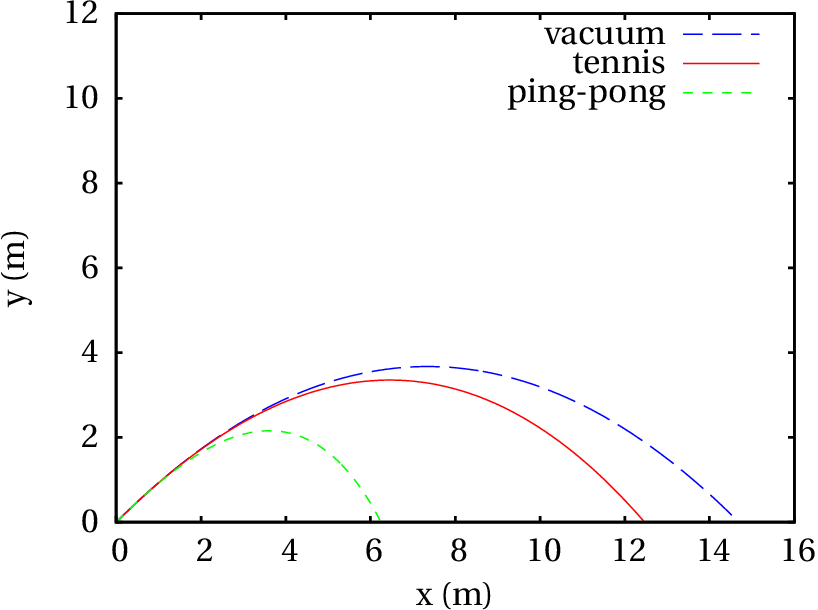Figure 10.8: Trajectories of a ball in vacuum, a tennis ball in air and a ping-pong ball in air.

The trajectories of the balls in the air are not parabolas because they curve more at the end, ending with a more vertical drop. The effect of air resistance is more visible on the ping-pong ball; although it is smaller than the tennis ball, the air resistance slows it more, due to its lower density. Launched with the same velocity, the horizontal ranges of the ping-pong and tennis balls are 6.2 m and 12.4 m. The hypothetical horizontal range of the two balls, if air resistance could be eliminated, would be 14.7 m.

### 10.4.3. Wilberforce pendulum

A Wilberforce pendulum (Fig. 10.9) consists of a cylinder that hangs from a very long vertical spring. When a spring is stretched or compressed, each turn changes slightly in size; in the Wilberforce pendulum, the large number of turns in the spring makes this change more visible; thus, as the spring oscillates up and down, it also winds or unwinds leading to rotation of the cylinder around its vertical axis.

This system has two degrees of freedom, the height of the center of mass of the cylinder and the angle of rotation of the cylinder, . If and are set in the equilibrium position, it is possible to ignore the gravitational potential energy that can be eliminated from the equations with a change of variables (see problem 4 in Chapter 9). The elastic potential energy has 3 terms, which depend on the elongation of the spring and its angle of rotation . The kinetic and potential energies are,

(10.20)

where , and are elastic constants of the spring. Lagrange equations, ignoring air resistance and other dissipative forces, lead to the following equations of motion:

(10.21)

To solve the evolution equations numerically, it is necessary to give some typical values ​​for the mass, the moment of inertia and the elastic constants,

(%i33) [m, I, k, a, b]: [0.5, 1e-4, 5, 1e-3, 0.5e-2]\$

The solution in the time interval from 0 to 40, with initial conditions cm and the other variables equal to 0, is obtained with the following command:

(%i34) sol: rk(['v,w,-(k*z+b*ang)/m,-(a*ang+b*z)/I], [z,ang,'v,w],[0.1,0,0,0],[t,0,40,0.01])\$

Figure 10.10 shows the plot obtained for the angle and the elongation , multiplied by a factor of 100 so that it is visible on the same scale of the angle.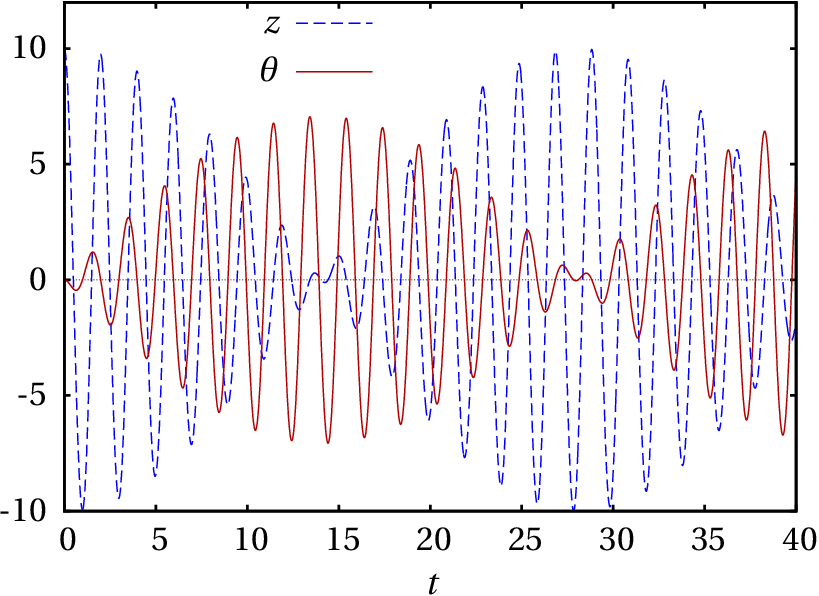Figure 10.10: Elongation and angle of rotation in a Wilberforce pendulum.

The graph shows an interesting feature of the Wilberforce pendulum: if the pendulum is made to oscillate without rotating, the amplitude of the linear oscillation decreases gradually, while the cylinder begins to rotate with torsional oscillation that reaches a maximum amplitude when the cylinder stops moving vertically. The amplitude of the torsional oscillation then begins to diminish as the linear oscillation grows again. This intermittence between vertical displacement and rotation repeats itself indefinitely.

The projection of the phase portrait in the and plane is shown in Figure 10.11.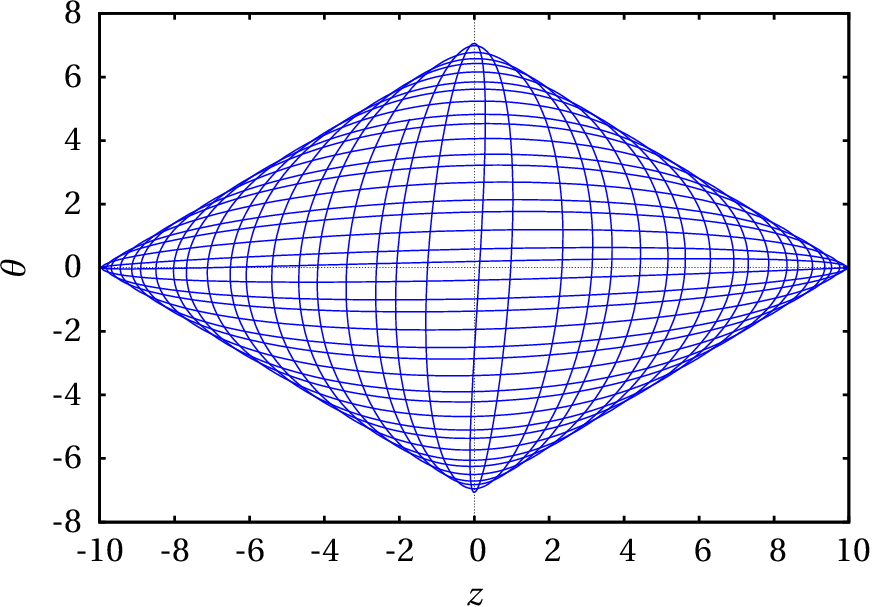Figure 10.11: Phase portrait on the plane formed by elongation and angle.

This system has two angular frequencies. The longitudinal angular frequency and the torsion angular frequency,

(10.22)

The cylinder in a Wilberforce pendulum usually has four nuts that can be displaced, increasing or decreasing the moment of inertia, to make the two frequencies very similar and the alternating effect between linear and rotational oscillations more visible. The values that we used for the parameters were chosen to guarantee two equal frequencies.

## Questions

1. The approximate value of the period of a pendulum with length is , where is the acceleration of gravity. This expression is a good approximation only in some situations. If the angle is zero at the stable equilibrium point, which of the following conditions guarantees that this expression is a good approximation of its real value?

1. angular velocity small.
2. acceleration of gravity small.
3. length small.
4. maximum value of small.
5. air resistance negligible.
2. If represents the speed of a particle and its position along its trajectory, the expression of the total tangential force on the particle is . How many equilibrium points does this system have?
1. 1
2. 2
3. 3
4. 4
5. 0
3. Which of the following is the Jacobian matrix of the system with evolution equations , ?
4. The evolution equations of a dynamical system with phase space ( , ), are , . Which of the following vectors has the same direction as the phase velocity in (1, 2)?
5. Regarding the phase portrait shown in the figure, what kind of equilibrium point is (1, 0)?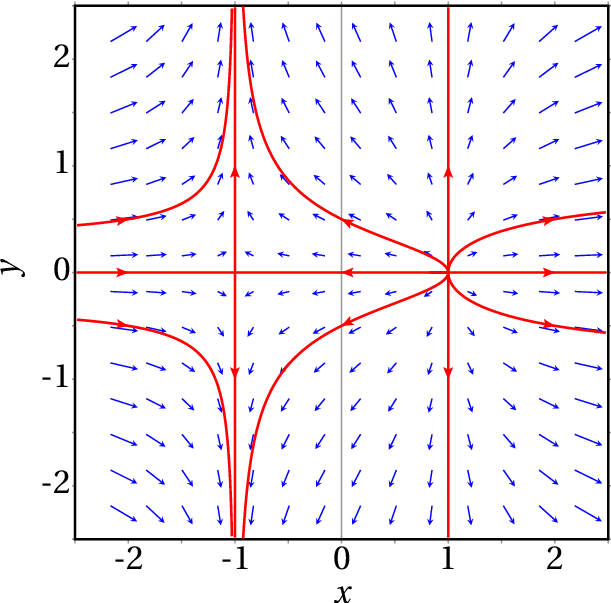1. attractive knot
2. repulsive focus
4. attractive focus
5. repulsive knot

## Problems

1. A particle of mass , moves along the x-axis under the action of a total force which depends on the position and the velocity . For each of the following cases find the equilibrium points, say what kind of points they are (stable or unstable, center, focus, knot or saddle) and plot the phase portrait showing the most important curves:
(a)
(b)
2. In each of the following cases find the equilibrium points and eigenvalues ​​of the Jacobian matrix at those points, and classify each of the equilibrium points:
(a)
(b)
(c)
(d)
3. The following plot shows the phase portrait of a system with only 3 points of equilibrium, in the idealized case where there is no friction. Sketch by hand the plot of the potential energy and the modified phase portrait when friction forces are considered.4. The angular amplitude of a swinging pendulum decreases, due to air resistance and friction in the axis. Consider a pendulum of length cm and mass kg, in which the friction in the axis can be neglected but the air resistance not. The equation of motion is Equation 8.8
If most of the mass is concentrated in a sphere of radius cm, the expression for the constant is given by Equation 4.14: , where  kg/m3 is the density of the air. Plot the graphs of , ; plot the evolution curve in phase space and explain its physical meaning in the following two cases:
(a) The pendulum is released from rest at an initial angle .
(b) The pendulum is released from , with initial angular velocity s-1.
5. The base of the pendulum of Figure 10.2 rotates in the horizontal plane, with constant angular velocity , while the pendulum swings.
(a) Show that the equation of motion is:
where is the distance from the center of mass to the axis and the effective length equals the square of the gyration radius, divided by .
(b) Plot the graph of as a function of , between and , for a pendulum with m and s-1. Repeat the plot by changing the value of to 8 s-1. Based on the two plots, find in each case the position of the stable and unstable equilibrium points.
(c) Show that when , there is a single stable equilibrium point at and a single unstable equilibrium point at .
(d) If , show that the equilibrium points at and are both unstable and two more stable equilibrium points appear at , where is an angle between zero and .
6. In the trajectory of the ping-pong ball obtained in section 10.4.2 , the horizontal range of the ball is approximately the value of the coordinate of the last point in list r1. Repeat the calculations changing the angle of the initial velocity above the horizontal; use the values 35°, 36°, 37°, 38°, 39°, and 40°. Make a table with the values of the horizontal range for each of those angles. From that table, determine the angle that leads to the biggest horizontal range. From the result of Problem 12 in Chapter 6, show that in vacuum the angle that produces the maximum range is 45°.
7. To analyze the nonlinear differential equation ,
(a) Write the evolution equations of the dynamical system equivalent to the equation.
(b) Find the equilibrium points of the system.
(c) Find the Jacobian matrix.
(d) Classify each of the equilibrium points.
(e) If in the values ​​of and its derivative are and , determine (numerically) the values ​​of the variable and its derivative at .
8. The dynamical system with evolution equations:
has a single equilibrium point at the origin. The Jacobian matrix at that point is equal to zero and therefore the (null) eigenvalues ​​can not be used to classify that equilibrium point. Use the following method to analyze the phase portrait of the system:
(a) Find the unit vector in the direction of the phase velocity at points on the axis and at points on the axis.
(b) Find the unit vector in the direction of the phase velocity at points on the two lines and .
(c) Sketch by hand the unit vectors found in the previous items at several points on the 4 quadrants of phase space, and plot some evolution curves following those phase velocity directions. On the basis of that plot, what kind of equilibrium point you think the origin is?
(d) Draw conclusions about the existence of cycles, homoclinic orbits or heteroclinic orbits and, if they exist, how many.
9. A particle of mass moves on the plane under the action of a conservative force with potential energy,
where and are two positive constants. The trajectories of the particle obtained with different values ​​of these constants are called Lissajous figures.
(a) Find the two equations of motion for and .
(b) Solve numerically the equations of motion, in the case , and (SI units), between and , if the particle starts from point (1, 0) with initial velocity . Plot its trajectory on the plane.
(c) Repeat the previous item, but now with the particle starting from (1, 0) with initial velocity .
(d) Note that the system can be considered as a set of two independent harmonic oscillators, in the directions and . Compute the oscillation period for each of those two oscillators and find the ratio between them.
(e) Repeat the procedure in item c, changing the value of to 18. What relationship do you see among the trajectories and the ratio ?
10. Any celestial body (planet, comet, asteroid, space probe, etc.) of mass in the solar system has a gravitational potential energy produced by the Sun, which is responsible for the elliptical orbits of these bodies. The expression for the potential energy is
where is the universal gravitational constant, is the mass of the Sun and the coordinates and are measured on the plane of the orbit of the celestial body, with the Sun qt the origin. If distances are measured in astronomical units, AU, and the time in years, the product will be equal to .
(a) Find the equations of motion of the celestial body in units of years for time and AU for distances.
(b) Comet Halley reaches a minimum distance from the Sun equal to 0.587 AU. At this point, its velocity is maximal, equal to 11.50 AU/year, and perpendicular to the segment from it to the Sun. Find numerically the orbit of Halley's comet, from the initial position , with initial velocity , using time intervals years. Plot the orbit from to years. What can you conclude about the numerical error?
(c) Repeat the procedure of the previous item with years and plot the orbit from to years. What can you conclude about the numerical error?
(d) Find the maximum distance that the Halley comet moves away from the Sun, and compare the comet's orbit with the orbits of the farthest planet, Neptune (orbit between 29.77 AU and 30.44 AU), and the closest planet to the Sun, Mercury (orbit between 0.31 AU and 0.39 AU). (Pluto is no longer considered a planet).

Questions: 1. D. 2. A. 3. C. 4. D. 5. E.

Problems

1. (a) Only a center at ( , ) = (0, 0).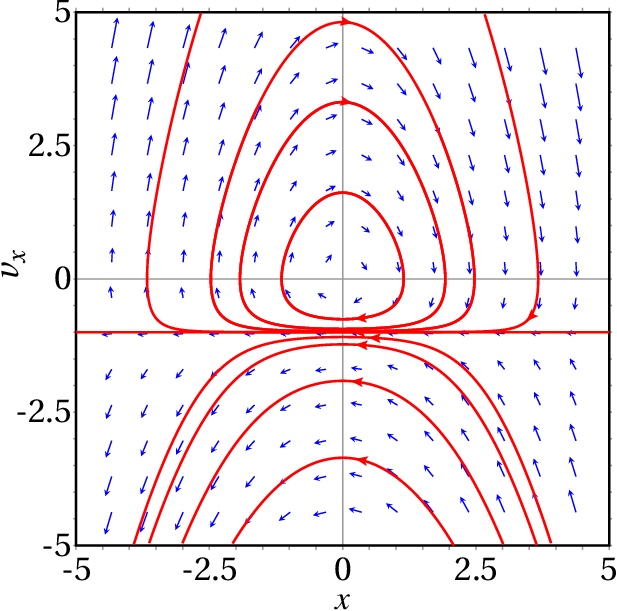(b) A saddle point at ( , ) = (0, 0), an unstable focus at ( , ) = (-1, 0) and a stable focus at ( , ) = (1, 0).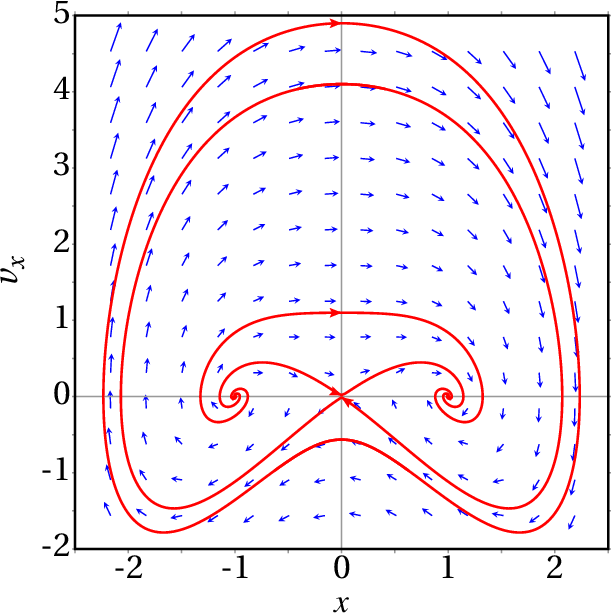2. (a) Two equilibrium points, both saddles: (3, -5), with eigenvalues ​​7 and -4, and (-4, 2) with eigenvalues ​​3 and -7.
(b) Two equilibrium points, (0, 0), with eigenvalues ​ is a center and (0.763, 0.874), with eigenvalues ​​-2.193 and 2.736 is a saddle.
(c) Two equilibrium points, ( , ) and ( , ), both saddles with eigenvalues .
(d) Nine equilibrium points. A saddle at (0, 0), with eigenvalues ​​3 and -5, other two saddles at ( , ) and ( , ), with eigenvalues ​​10 and -12, other two saddles at ( , ) and ( , ), with eigenvalues 4 and -6, and four attractive foci at ( , ), ( , ), ( , ) and ( , ), with eigenvalues , where and .
3. The saddle points remain saddle points and the center becomes a stable focus.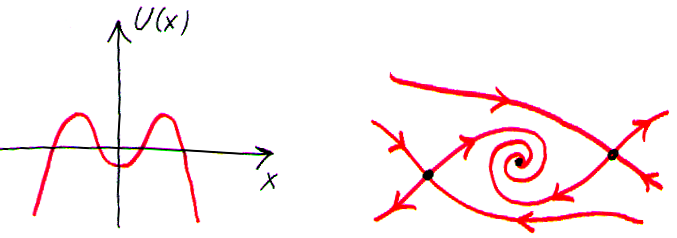4. (a) The pendulum oscillates with a slowly decreasing amplitude: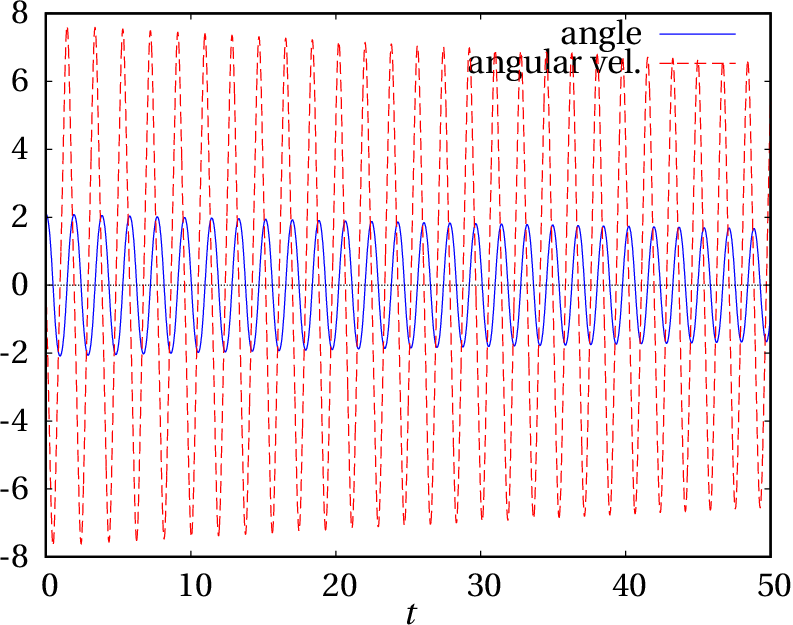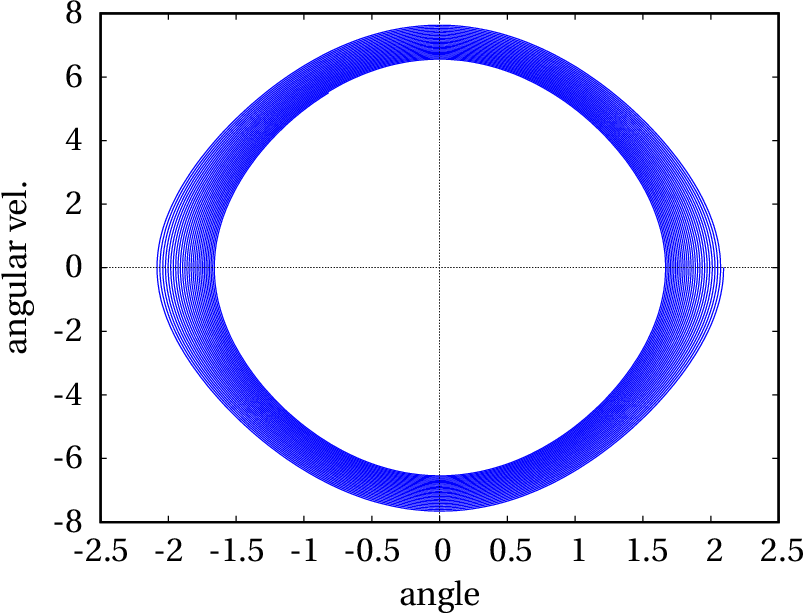(b) The pendulum does three full turns, turning clockwise, and when it passes the fourth time through the stable equilibrium position, it begins to oscillate with a slowly decreasing amplitude: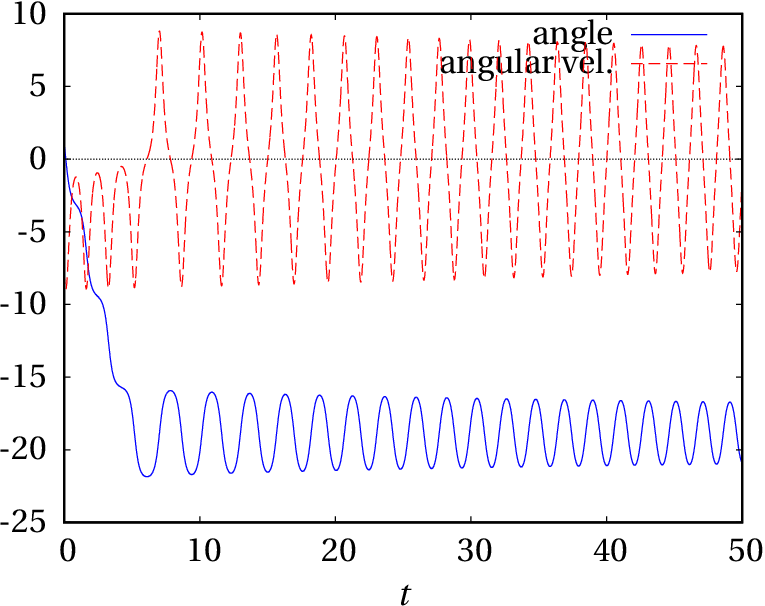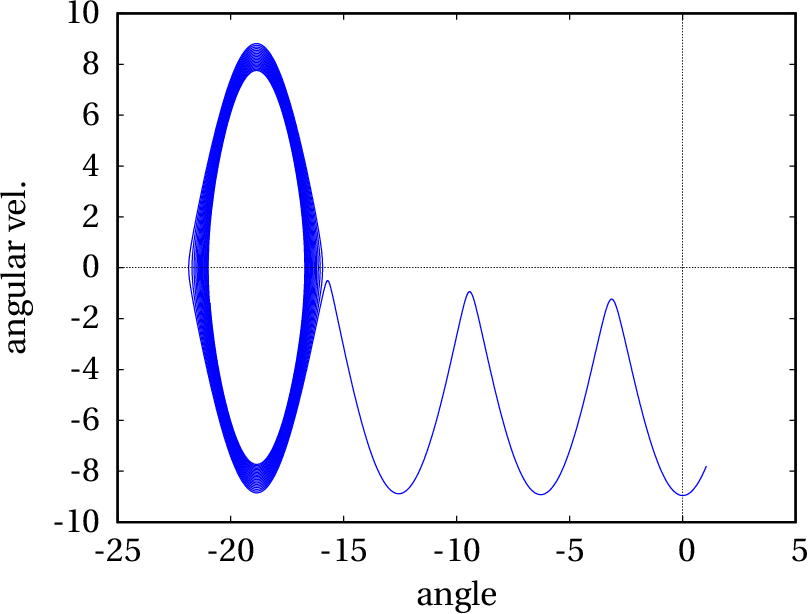5. (b) With s-1, there is a stable equilibrium point at and an unstable equilibrium point at . With s-1, there are two unstable equilibrium points at and and two stable equilibrium points at and .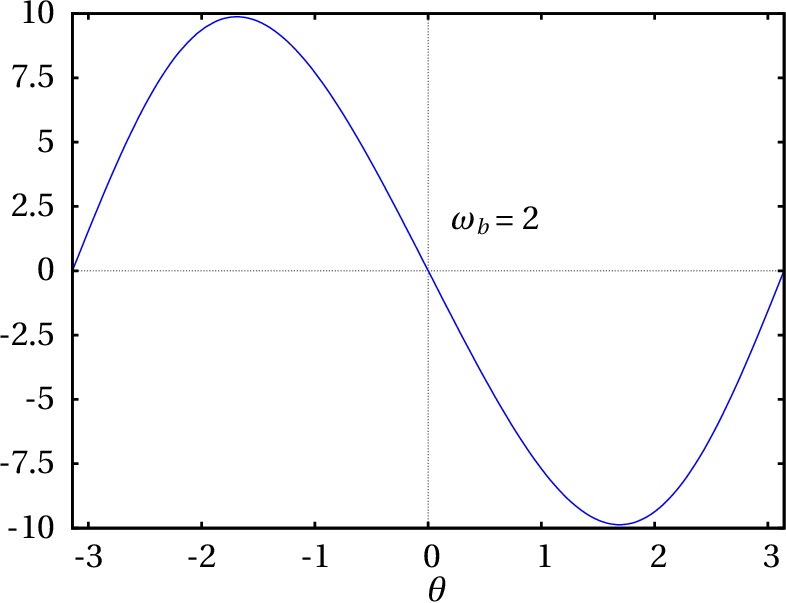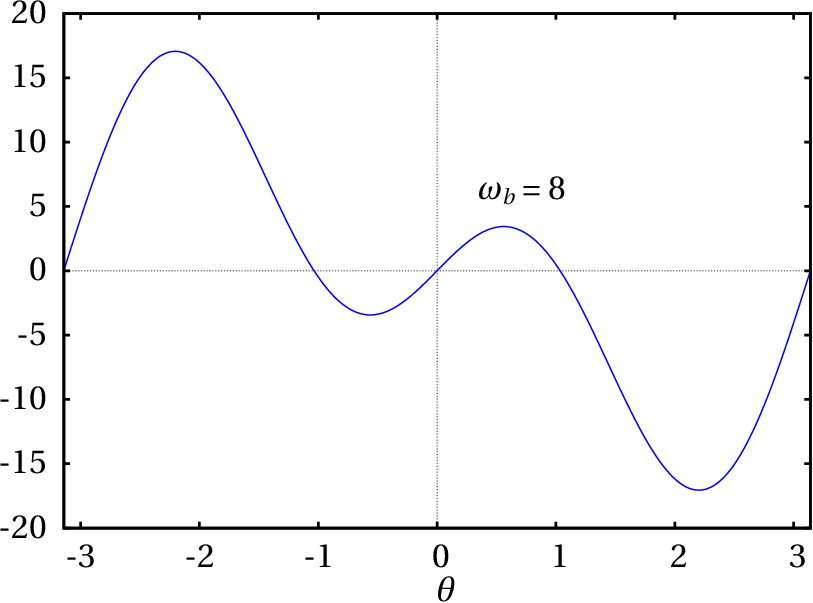6.  Angle Range (m) 35° 6.293 36° 6.299 37° 6.301 38° 6.299 39° 6.325 40° 6.314
The 37° angle produces the maximum range. In problem 12 of Chapter 6 , the maximum value of the sine is 1, when ; therefore, .
7. (a) ,   (b) ( , )=(1, 0) and ( , )=(-1, 0)   (c)   (d) (1, 0) is a center (-1, 0) is a saddle point.  (e) , .
8. (a) On the axis, . On the axis, . (b) On the line , . On the line , . (c) See the figure; the origin is a saddle. (d) There are no cycles or heteroclinic orbits; there is an infinite number of homoclinic orbits (all the evolution curves in the first and third quadrants).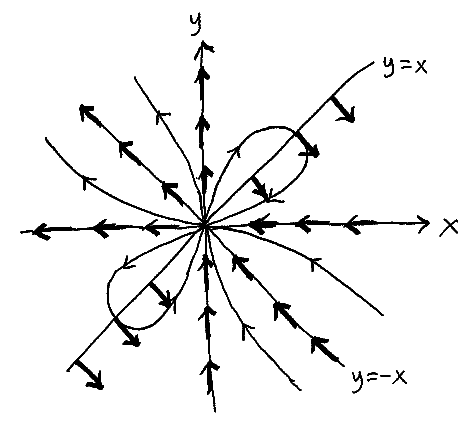9. (a)
(b) and (c)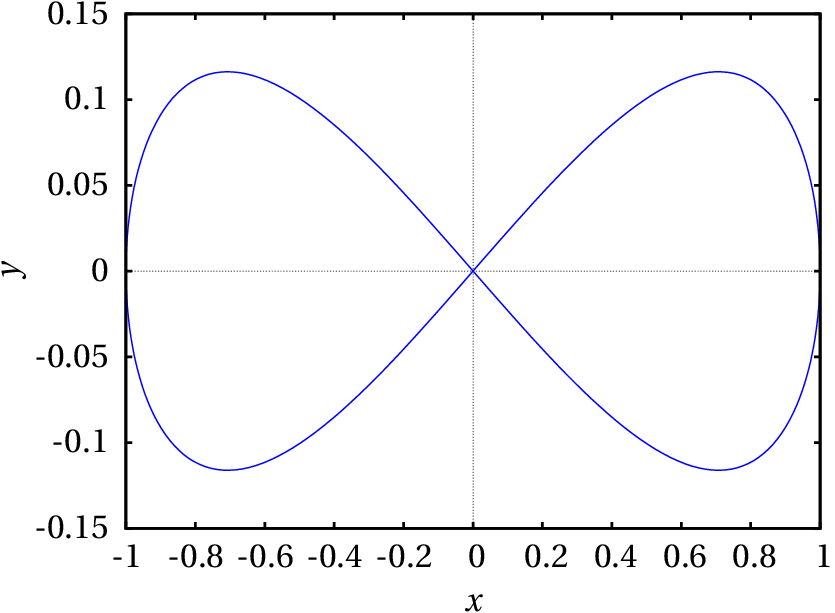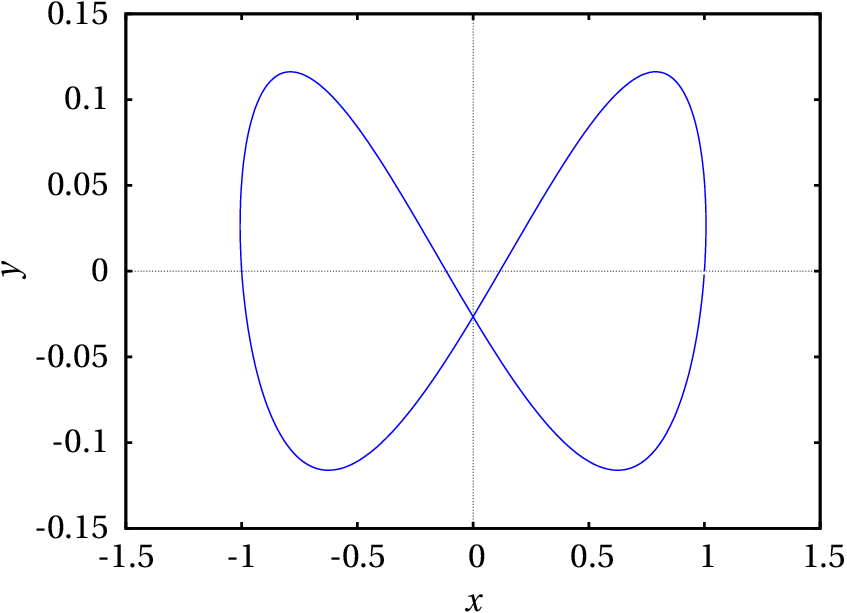(d) Along the direction, 2.433 s. Along the direction, 1.217 s. The period in the direction is twice the period in the direction. (e) If is an integer, the state of the particle returns to the initial state after describing a Lissajous figure with loops along the axis.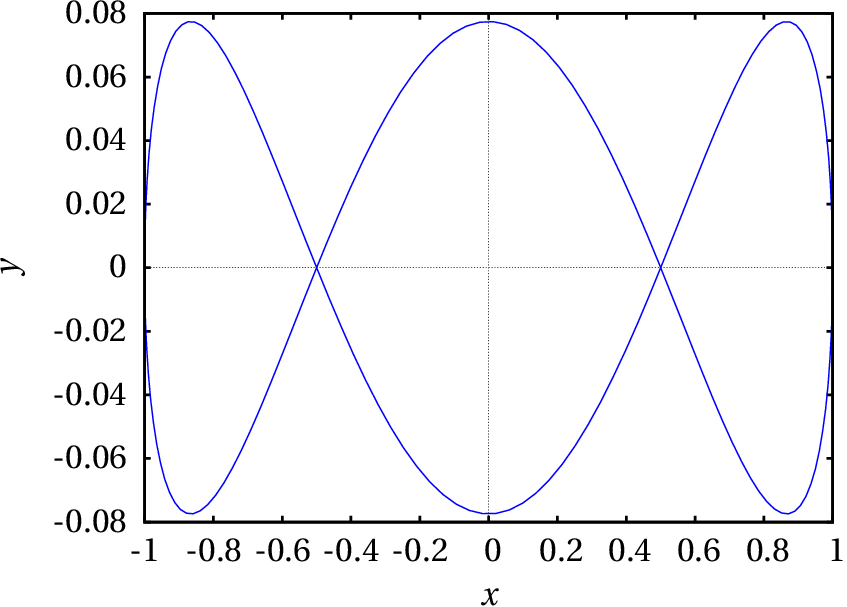10. (a)
(b) and (c)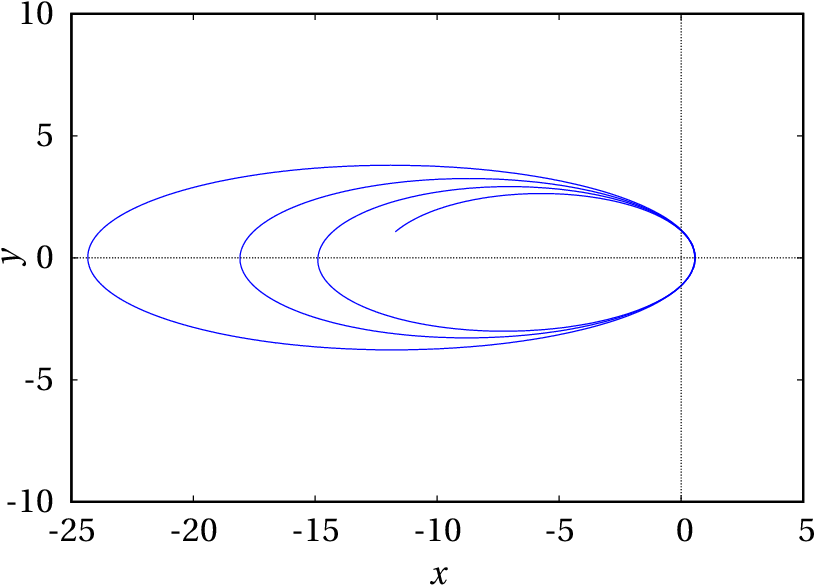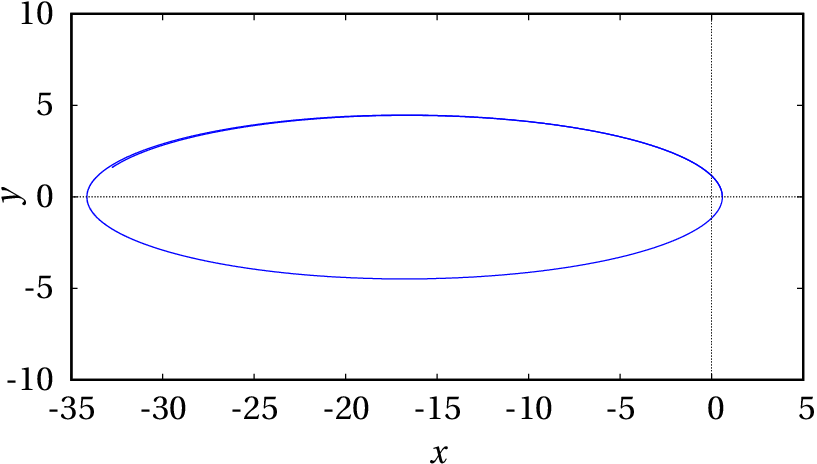In item (b), the numerical error is very high; the comet's energy does not remain constant but decreases. In (c) the numerical error is much smaller, but the comet continues to lose energy; it would be necessary to further reduce the value of to reduce the error. (d) 34.4 AU. The orbit exits out of Neptune's orbit, and enters a point between the orbits of Mercury and Venus.
Wrong

(click to continue)

Wrong

(click to continue)

Wrong

(click to continue)

Correct

(click to continue)

Wrong

(click to continue)

Correct

(click to continue)

Wrong

(click to continue)

Wrong

(click to continue)

Wrong

(click to continue)

Wrong

(click to continue)

Wrong

(click to continue)

Wrong

(click to continue)

Correct

(click to continue)

Wrong

(click to continue)

Wrong

(click to continue)

Wrong

(click to continue)

Wrong

(click to continue)

Wrong

(click to continue)

Correct

(click to continue)

Wrong

(click to continue)

Wrong

(click to continue)

Wrong

(click to continue)

Wrong

(click to continue)

Wrong

(click to continue)

Correct

(click to continue)